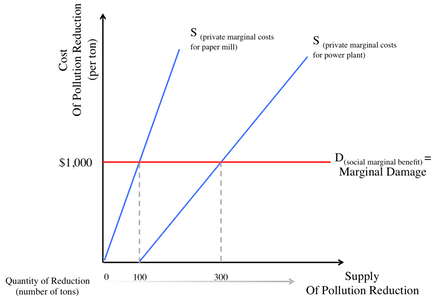Regulation Or Taxation?Suppose that there are two polluters in a city, both releasing $\ce{CO_2}$ into the atmosphere. One is a paper mill and the other is a power plant. The marginal damage from each ton of $\ce{CO_2}$ they produce is $\1,000$, but they have different marginal costs to reduce that pollution, as represented by the graph to the right.

What's the most efficient solution for the government to reduce 400 tons of $\ce{CO_2}$? To establish a tax of $\1,000$ per ton of $\ce{CO_2}$ produced? Or to be fair, establish a policy that forces each plant to separately cut 200 tons of $\ce{CO_2}$? Assume that either taxation or regulation is enforceable and that an efficient solution means economically efficient.

×